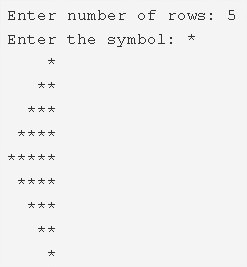# C Program Mirrored Half Diamond Star Pattern | C Patterns

C program to print a mirrored half diamond star pattern – In this article, we will brief in on the several ways to print a mirrored half diamond star pattern in C programming.

Suitable examples and sample programs have also been added so that you can understand the whole thing very clearly. The compiler has also been added with which you can execute it yourself.

The means used in this specific piece are as follows:

• Using For Loop
• Using While Loop
• Using Do-While Loop

As we all know, a diamond is a very common shape used in the field of geometry and life in general. The geometrical figure is much easier compared to the one in reality where the figure of the salary matters.

However, as per the name of the article, a mirrored half diamond star looks like this:As you can see, you need to particularise the number of rows to be mentioned. Subsequently, the mirrored half diamond pattern will be printed.

The number of rows mentioned is applied to both the directions from the centre of the diamond.

Thus, the multiple means that are discussed in this piece on how to print a mirrored half diamond star pattern are as follows:

## Using For Loop

1. Read the no.of rows, enter the character and store the values into the variables n, ch.

2) The 1st for loop iterates through rows with the structure for(i=1;i<=n;i++).

a)The 2nd for loop iterates through columns with the structure for(j=1;j<=n-i;j++).

It prints space.

b) The 3rd for loop iterates through columns with the structure for(j=1;j<=i;j++).

It prints symbol.

c) Cursor comes to next line for each iteration of i.

After completion of all iterations of i, the half part of the mirrored half diamond star pattern will be printed.

3) The 4th for loop iterates through rows with the structure for(i=n-1;i>0;i–).

a) The 5th for loop iterates through columns with the structure for(j=1;j<=n-1;j++)

It prints space.

b) The 6th for loop iterates through columns  with the structure for(j=1;j<=i;j++)

It prints symbol.

c) Cursor comes to next line for each iteration of i.

After all iterations of i, the remaining half part of the mirrored half diamond star pattern will be printed.

Output:

### Using While Loop

1. The while loop first checks the condition, if the condition is true, then only it executes the code.

2) The 1st while loop with the condition i<=n iterates through rows until the condition becomes false.

a) The 2nd while loop with the condition j<=n-i iterates through columns until the condition becomes false.

It prints space.j value increased by 1. The 2nd while loop again checks the condition.

b) The 3rd while loop with the condition j<=i iterates through columns until the condition becomes false.

It prints symbol.j value increased by 1. The 3rd loop again checks the condition.

c) Cursor comes to next line, i value increased by 1. The 1st while loop again checks the condition.

After the above steps, the half part of the mirrored half diamond will be printed.

3) i=n-1, The 4th while loop with the condition i>0 iterates through rows until the condition becomes false.

a) The 5th while loop iterates through columns until the condition j<=n-i becomes false.

It prints space.j value increased by 1. The 5th while loop again checks the condition.

b) The 6th while loop iterates through columns until the condition j<=i becomes false.

It prints symbol.j value increased by 1. The 6th while loop again checks the condition.

c) Cursor comes to next line.i value decreased by 1. The 4th loop again checks the condition.

After all iterations of the 4th while loop, the remaining half part of the mirrored half diamond will be printed.

Output:

#### Using Do-While Loop

1. i=1, The 1st do-while loop iterates through rows.

a) j=1, The 2nd do-while loop iterates through columns.

Prints space,j value increased by 1.

Checks the condition j<=n-i+1. If the condition is true then loop iterates again. Repeats until the condition becomes false.

b) j=1, The 3rd do-while loop iterates through columns.

It prints symbol.j value increased by 1.

Checks the condition j<=i. If the condition is true then loop iterates again. Repeats until the condition becomes false.

c) Cursor comes to next line. i value increased by 1. Checks the condition i<=n. If the condition is true then the loop iterates again. Repeats until the condition becomes false.

From the above steps, we will get first half part of the mirrored half diamond star pattern.

2) i=n-1 The 4th do-while loop iterates through rows.

a) The 5th do-while loop iterates through columns.

It prints space.j value increased by 1.

Checks the condition j<=n-i+1. If the condition is true then the loop iterates again. Repeats until the condition becomes false.

b) The 6th do-while loop iterates through columns.

It prints symbol.j value increased by 1.

Checks the condition j<=i. If the condition is true then loop iterates again. Repeats until the condition becomes false.

c) Cursor comes to next line.i value decreased by 1. Checks the condition. If the condition is true then loop iterates again. Repeats until the condition becomes false.

From the above steps, we will get remaining half part of the mirrored half diamond star pattern.

Output:
x

## C Program To Check If Alphabet, Digit or Special Character | C Programs

C program to check for an alphabet, digit or special character – In this article, ...